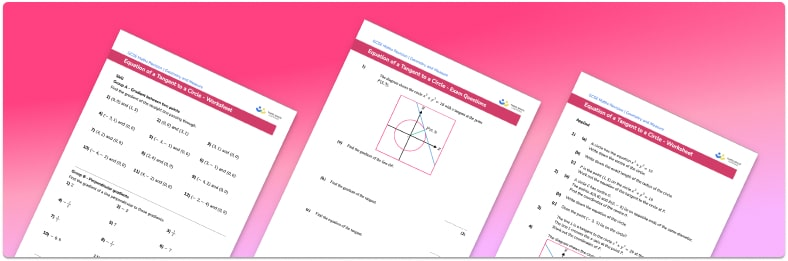# Equation of a tangent to a circle worksheetHelp your students prepare for their Maths GCSE with this free equation of a tangent to a circle worksheet of 30 + questions and answers

• Section 1 of the equation of a tangent to a circle worksheet contains 20+ skills-based equation of a tangent to a circle questions, in 3 groups to support differentiation
• Section 2 contains 3 applied equation of a tangent to a circle questions with a mix of worded problems and deeper problem solving questions
• Section 3 contains 3 foundation and higher level GCSE exam style equation of a tangent to a circle questions
• Answers and a mark scheme for all equation of a tangent to a circle questions are provided
• Questions follow variation theory with plenty of opportunities for students to work independently at their own level
• All questions created by fully qualified expert secondary maths teachers
• Suitable for GCSE maths revision for AQA, OCR and Edexcel exam boards

• This field is for validation purposes and should be left unchanged.

You can unsubscribe at any time (each email we send will contain an easy way to unsubscribe). To find out more about how we use your data, see our privacy policy.

### Equation of a tangent to a circle at a glance

The tangent to a circle is a straight line that touches a curve at a single point. The distance from the centre of the circle (sometimes referred to a centre O) to the circumference of the circle is called the radius.

The radius of the circle and the tangent are perpendicular lines as they meet at a right angle. In order to find the equation of the tangent you need to find the gradient of the tangent which can be calculated by working out the reciprocal of the gradient of the line that intersects it at a right angle (the gradient of the radius).

Then you need to find the y intercept by substituting in the coordinates of the point where the tangent and radius intersect.

Looking forward, students can then progress to additional number worksheets, for example a Area and circumference of a circle worksheet or a Circles, Arcs And Sectors worksheet.For more teaching and learning support on Geometry our GCSE maths lessons provide step by step support for all GCSE maths concepts.

## Do you have KS4 students who need more focused attention to succeed at GCSE?There will be students in your class who require individual attention to help them succeed in their maths GCSEs. In a class of 30, it’s not always easy to provide.

Help your students feel confident with exam-style questions and the strategies they’ll need to answer them correctly with our dedicated GCSE maths revision programme.

Lessons are selected to provide support where each student needs it most, and specially-trained GCSE maths tutors adapt the pitch and pace of each lesson. This ensures a personalised revision programme that raises grades and boosts confidence.

Find out more